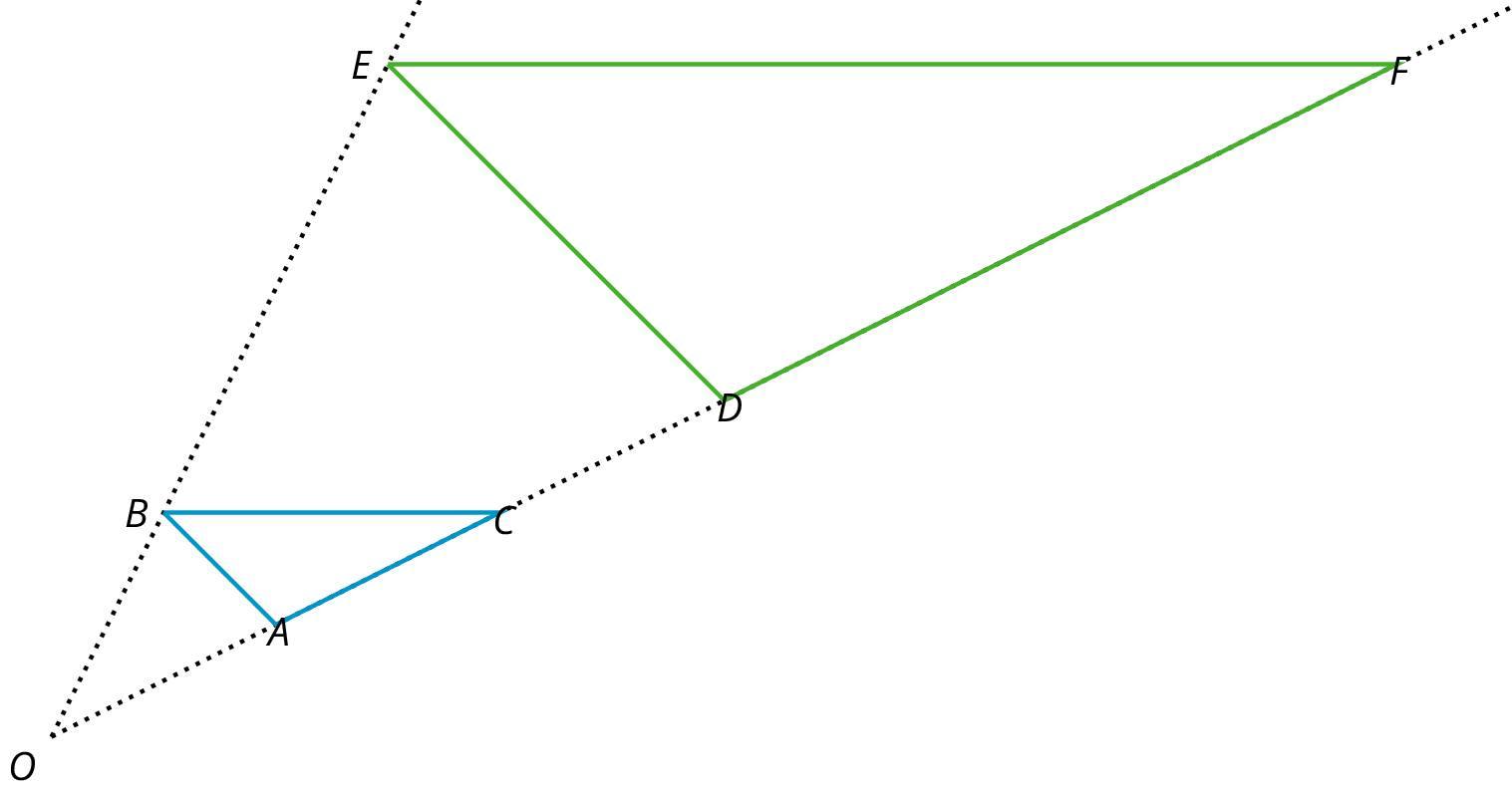# Lesson 10

Dilations on a Square Grid

Let’s dilate figures on a square grid.

### 10.1: Dilations on a Grid

1. Find the dilation of triangle $$QRS$$ with center $$T$$ and scale factor 2.
2. Find the dilation of triangle $$QRS$$ with center $$T$$ and scale factor $$\frac{1}{2}$$.

### 10.2: Card Sort: Matching Dilations on a Coordinate Grid

Your teacher will give you some cards. Each of Cards 1 through 6 shows a figure in the coordinate plane and describes a dilation.

Each of Cards A through E describes the image of the dilation for one of the numbered cards.

Match number cards with letter cards. One of the number cards will not have a match. For this card, you’ll need to draw an image.

The image of a circle under dilation is a circle when the center of the dilation is the center of the circle. What happens if the center of dilation is a point on the circle? Using center of dilation $$(0,0)$$ and scale factor 1.5, dilate the circle shown on the diagram. This diagram shows some points to try dilating.

### 10.3: Info Gap: Dilations

Your teacher will give you either a problem card or a data card. Do not show or read your card to your partner.

If your teacher gives you the problem card:

3. Explain how you are using the information to solve the problem.

Continue to ask questions until you have enough information to solve the problem.

4. Share the problem card and solve the problem independently.

If your teacher gives you the data card:

2. Ask your partner “What specific information do you need?” and wait for them to ask for information.

If your partner asks for information that is not on the card, do not do the calculations for them. Tell them you don’t have that information.

3. Before sharing the information, ask “Why do you need that information?” Listen to your partner’s reasoning and ask clarifying questions.

4. Read the problem card and solve the problem independently.

5. Share the data card and discuss your reasoning.

Triangle $$EFG$$ was created by dilating triangle $$ABC$$ using a scale factor of 2 and center $$D$$. Triangle $$HIJ$$ was created by dilating triangle $$ABC$$ using a scale factor of $$\frac12$$ and center $$D$$.

1. What would the image of triangle $$ABC$$ look like under a dilation with scale factor 0?
2. What would the image of the triangle look like under dilation with a scale factor of -1? If possible, draw it and label the vertices $$A’$$, $$B’$$, and $$C’$$. If it’s not possible, explain why not.

3. If possible, describe what happens to a shape if it is dilated with a negative scale factor. If dilating with a negative scale factor is not possible, explain why not.

### Summary

Square grids can be useful for showing dilations. The grid is helpful especially when the center of dilation and the point(s) being dilated lie at grid points. Rather than using a ruler to measure the distance between the points, we can count grid units.

For example, suppose we want to dilate point $$Q$$ with center of dilation $$P$$ and scale factor $$\frac{3}{2}$$. Since $$Q$$ is 4 grid squares to the left and 2 grid squares down from $$P$$, the dilation will be 6 grid squares to the left and 3 grid squares down from $$P$$ (can you see why?). The dilated image is marked as $$Q’$$ in the picture.

Sometimes the square grid comes with coordinates. The coordinate grid gives us a convenient way to name points, and sometimes the coordinates of the image can be found with just arithmetic.

For example, to make a dilation with center $$(0,0)$$ and scale factor 2 of the triangle with coordinates $$(\text-1, \text- 2)$$, $$(3,1)$$, and $$(2, \text- 1)$$, we can just double the coordinates to get $$(\text- 2, \text- 4)$$, $$(6,2)$$, and $$(4, \text- 2)$$.

In general, an important use of coordinates is to communicate geometric information precisely. Let’s consider a quadrilateral $$ABCD$$ in the coordinate plane. Performing a dilation of $$ABCD$$ requires three vital pieces of information:

1. The coordinates of $$A$$, $$B$$, $$C$$, and $$D$$
2. The coordinates of the center of dilation, $$P$$
3. The scale factor of the dilation

With this information, we can dilate the vertices $$A$$, $$B$$, $$C$$, and $$D$$ and then draw the corresponding segments to find the dilation of $$ABCD$$. Without coordinates, describing the location of the new points would likely require sharing a picture of the polygon and the center of dilation.

### Glossary Entries

• center of a dilation

The center of a dilation is a fixed point on a plane. It is the starting point from which we measure distances in a dilation.

In this diagram, point $$P$$ is the center of the dilation.

• dilation

A dilation with center $$O$$ and positive scale factor $$r$$ takes a point $$P$$ along the line $$OP$$ to another point whose distance is $$r$$ times further away from $$O$$ than $$P$$ is. If $$r < 1$$ then the new point is really closer to $$O$$, not further away.

The triangle $$DEF$$ is a dilation of the triangle $$ABC$$ with center $$O$$ and with scale factor 3. So $$D$$ is 3 times further away from $$O$$ than $$A$$ is, $$E$$ is 3 times further away from $$O$$ than $$B$$ is, and $$F$$ is 3 times further away from $$O$$ than $$C$$ is.• scale factor

To create a scaled copy, we multiply all the lengths in the original figure by the same number. This number is called the scale factor.

In this example, the scale factor is 1.5, because $$4 \boldcdot (1.5) = 6$$, $$5 \boldcdot (1.5)=7.5$$, and $$6 \boldcdot (1.5)=9$$.